### Sets And Functions Class 10th Mathematics Tamilnadu Board Solution

##### Question 1.State whether each of the following arrow diagrams define a function or not. Justify your answer.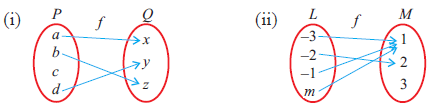Answer:If P and Q are two relation then the two most important conditions for the relation to be treated as a function are as follows,● Each element of P must have a unique image in Q.● Each element of P has to be mapped with only one element in QIf either of the above conditions is not satisfied, then the relation can’t be consider as a function.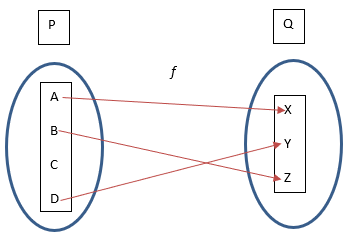(i) The figure shows mapping from P to Q, the element "C" in P does not have any image in Q. Since, the above given relation does not meet the conditions for relation and functions mentioned above so it is not a function.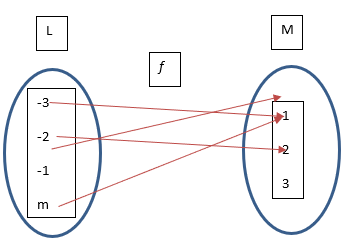(ii) In the above mapping from L to m, each element of L has an image in M and the number of image for each element is one. Since, the above given relation satisfies all the conditions of relation and function mentioned above, so it is a function.Question 2.For the given function F = { (1, 3), (2, 5), (4, 7), (5, 9), (3, 1) }, write the domain and range.Answer:We know that for any two non-empty sets A and B. A function f from A to B may be defined as a rule that assigns each element of set A to tha of set B in a unique way (∀ x ∈ A and y ∈ B).We denote y = f(x) which means mean y is a function of x. so the set A is called the domain of the function and set B is called the co-domain of the function. y is called as the image of x under f and x is called a preimage of y. Range may be defined as the set of all image and it’s a subset of co-domain.We can represent the function in set as described belowf = {(a,b) / a ∈ A and b ∈ B}Where A is the domain and B is the pre-domain.So as per data given,F = {(1, 3), (2, 5), (4, 7), (5, 9), (3, 1)}HereDomain = {1, 2, 3, 4, 5}Range = {1, 3, 5, 7, 9}Question 3.Let A = { 10, 11, 12, 13, 14 }; B = { 0, 1, 2, 3, 5 } and fi : A → B , i = 1,2,3.State the type of function for the following (give reason):(i) f1 = { (10, 1), (11, 2), (12, 3), (13, 5), (14, 3) }(ii) f2 = { (10, 1), (11, 1), (12, 1), (13, 1), (14, 1) }(iii) f3 = { (10, 0), (11, 1), (12, 2), (13, 3), (14, 5) }Answer:There are several kind of function such as,One-one function:If f : A → B is a function then its treated as an one to one function if no element of B is associated with more than one element of A . A one-one function is also called an injective function.Onto function:A function f:A→B is called as an onto function if every element in B has a pre-image in A.One-one and onto function:A function f:A→B is called as an One-one and onto function if f maps all distinct elements of A with all distinct images in B and all element in B is an image of some element in A.Constant function:A function f:A→B is called as Constant function if all distinct elements of A has a single image in B.Here we are givenA = { 10, 11, 12, 13, 14 }B = { 0, 1, 2, 3, 5 }fi:A → B , where i = 1,2,3.(i) Here both 12 and 14 have same image i.e. 3 and there is no preimage of 0 of B in A, So it’s clear that the function given is neither an one to one nor onto.(ii) Here it’s clear that for all element of A there is only one image in B i.e. 1.we can also write that for f2:A→ B, f2(x) = 1, ∀ x ∈ 1. So the above function is a constant function.(iii) Here range of f3 = {0,1,2,3,4,5} = BIt’s an one-one and onto function as all element in A have a distinct and non-repetitive image in BQuestion 4.If X = {1, 2, 3, 4, 5 }, Y = { 1, 3, 5, 7, 9 } determine which of the following relations from X to Y are functions? Give reason for your answer. If it is a function, state its type.(i) R1 = {(x, y)| y = x + 2, x ∈ X , y ∈ Y }(ii) R2 = {(1, 1), (2, 1), (3, 3), (4, 3), (5, 5)}(iii) R3 = {(1, 1), (1, 3), (3, 5), (3, 7), (5, 7)}(iv) R4 = {(1, 3), (2, 5), (4, 7), (5, 9), (3, 1)}Answer:Here we are givenX = {1, 2, 3, 4, 5}, Y = { 1, 3, 5, 7, 9 }(i) R1 = {(x, y)| y = x + 2, x ∈ X , y ∈ Y }So using the value we can write the function in set formR1 = {(1,3),(3,5),(5,7)}From the above form it’s clear that as the elements of X doesn’t have a unique image in Y so it’s not a function.(ii) R2 = {(1, 1), (2, 1), (3, 3), (4, 3), (5, 5)}Here R2 is a function as all the element of X has a distinct image in Y. As 1 and 2 of X is related to 1 of Y and 3, 4 of X is related to 3 of Y so this function is onto function.(iii) R3 = {(1, 1), (1, 3), (3, 5), (3, 7), (5, 7)}Here in this relation 1 of X is related to 1 and 3 of Y which clearly contradict the definition of a function which says that every elements of a domain should have at most one image. So it’s clear that it’s not a function.(iv) R4 = {(1, 3), (2, 5), (4, 7), (5, 9), (3, 1)}The above expression is a onto and one-one function as all the elements of X has a unique image in Y and no two elements of X have same image in Y. these functions are also termed as bijective function.Question 5.If R = {(a, - 2), (- 5, b), (8, c), (d, - 1)} represents the identity function, find the values of a, b, c and d.Answer:Identity function is a function given by f(x) = x.Given function: R = {(a, - 2), (- 5, b), (8, c), (d, - 1)}This means,f(a) = -2f(-5) = bf(8) = cf(d) = -1Now, from the definition, f(x) = xtherefore,a = -2-5 = b, b = -58 = c, c = 8d = -1So a = -2, b = -5, c = 8 and d = -1Question 6.A = {–2, –1, 1, 2 } and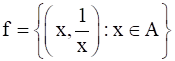. Write down the range of f. Is f a function from A to A?Answer:From the elements of A we can write the function in set orderSo f =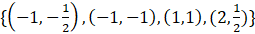Here from the above expression the range of the function is given asRange of f =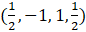As the element of the range are not in the set A so clearly it’s not a function from A to A.Question 7.Let f = {(2, 7), (3, 4), (7, 9), (–1, 6), (0, 2), (5, 3) } be a function from A = { –1, 0, 2, 3, 5, 7 } to B = { 2, 3, 4, 6, 7, 9}.Answer:Here we are given thatf = {(2, 7), (3, 4), (7, 9), (–1, 6), (0, 2), (5, 3)}A = { –1, 0, 2, 3, 5, 7 }B = { 2, 3, 4, 6, 7, 9 } where f:A→ BHere from the above expression the range of the function can be given asRange of f = {7, 4, 9, 6, 2, 3} = BSo it clear that it is an onto function, again the mapping shows that every element of A is mapped to an unique element of B and no two elements of A is linked with a single element of B so it’s a one-one function too.Hence its clear that the expression is a one-one and onto functionQuestion 8.Write the pre-images of 2 and 3 in the functionf = { (12, 2), (13, 3), (15, 3), (14, 2), (17, 17) }.Answer: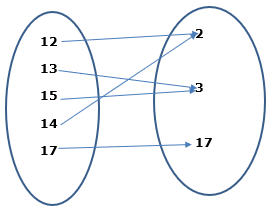So from the image we can see thatPre-image of 2 are 12 and 14Pre-image of 3 are 13 and 15Question 9.The following table represents a function from A = { 5, 6, 8, 10 } to B = { 19, 15, 9, 11 } where f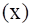= 2x - 1. Find the values of a and b.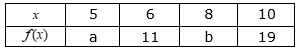Answer:It’s given that f(x) = 2x-1So f(5) = 2 × 5 -1= 10-1= 9∴ a = 5Similarly f(8) = 2 × 8 -1= 16-1= 15∴ b = 15So the values of a = 9 and b = 15Question 10.Let A = {5, 6, 7, 8 }; B = { –11, 4, 7, –10,–7, –9,–13 } and f = {( x,y) : y = 3 - 2x, x ∈ A, y ∈ B }|(i) Write down the elements of f. (ii) What is the co-domain?(iii) What is the range? (iv) Identify the type of function.Answer:Here we are givenA = {5, 6, 7, 8 }B = {–11, 4, 7, –10,–7, –9,–13 }f = {( x,y) : y = 3 - 2x, x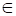A, yB }so we can findf = {( x,y) : y = 3 - 2x, xA, yB }f(5) = 3- 2×5 = 3-10 = -7f(6) = 3 – 2×6 = 3-12 = -9f(7) = 3 – 2×7 = 3-14 = -11f(8) = 3 – 2×8 = 3- 16 = -13(i) the elements of f areFrom the above expression we get thatf = {(5,-7),(6,-9),(7,-11),(8,-13)}(ii) co-domainThe co-domain is B and it can be given asCodomain B = {-11,4, 7,-10,-7,-9,-13}(iii) RangeRange may be defined as the image of A in B present in the function.Range of f = {-7,-9,-11,-13}(iv) type of function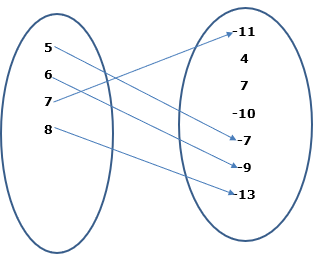From the above data and the diagram it’s clear that the given function is a one-one function as all the element A has a unique and single image in B. it’s not a onto function as the range is not same as the co-domain .Question 11.State whether the following graphs represent a function. Give reason for your answer.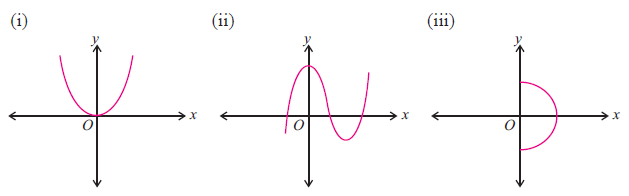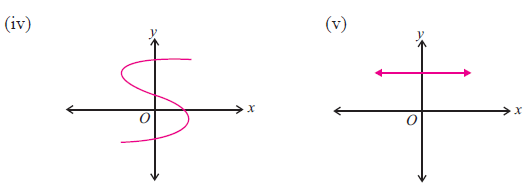Answer:(i)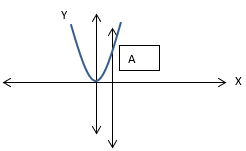The above graph represents a function as we know that a graph represents a function only when the curve in the graph cuts the vertical axis only at a single point A.Here the curve cuts the vertical line at point X1 so it’s a function.(ii)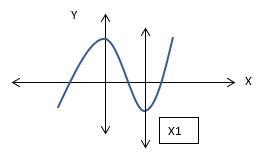The above graph represents a function as a vertical line cuts the curve at X1 point.(iii)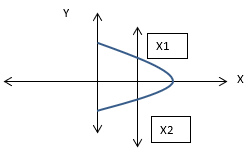The above graoh doesn’t represents a function as the vertical line and the curve intersects ech other at two points X1 and X2.(iv)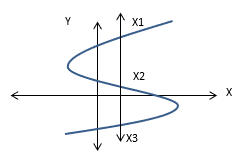The above figure doesn’t represent a function as the vertical line cuts the curve at three different points,.(v)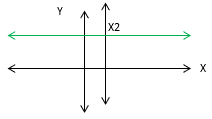The graph is are presentation of a function as the straight line is cut at a single point X2 by the vertical line.Question 12.Represent the function f = { (–1, 2), (– 3, 1), (–5, 6), (– 4, 3) } as(i) a table (ii) an arrow diagramAnswer:We are givenf = { (–1, 2), (– 3, 1), (–5, 6), (– 4, 3) }(i) a tableRepresenting the given data in tabular form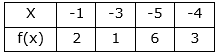(ii) an arrow diagramwe are givenA = {-1, -3, -5, -4}B = {2, 1, 6, 3}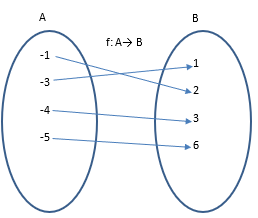Question 13.Let A = { 6, 9, 15, 18, 21 }; B = { 1, 2, 4, 5, 6 } and f : A → B be defined by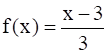. Represent f by(i) an arrow diagram(ii) a set of ordered pairs(iii) a table(iv) a graph.Answer:Given A = { 6, 9, 15, 18, 21 }B = { 1, 2, 4, 5, 6 } and f : A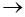BAnd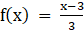We can find the domain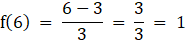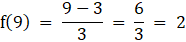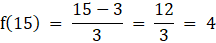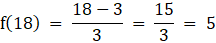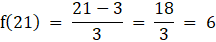(i) an arrow diagram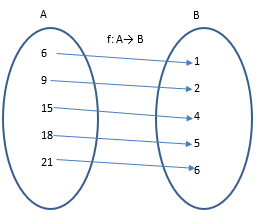(ii) a set of ordered pairsFrom the given data and function value we can write the function as set of order pair as followsf(x) = {(6,1),(9,2),(15,4),(18,5),(21,6)}(iii) a tableFrom the data we can draw the tabular representation taking X and f(X)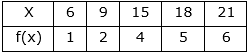(iv) a graph.We can represent the function values in graph as followsf(x) = {(6,1),(9,2),(15,4),(18,5),(21,6)}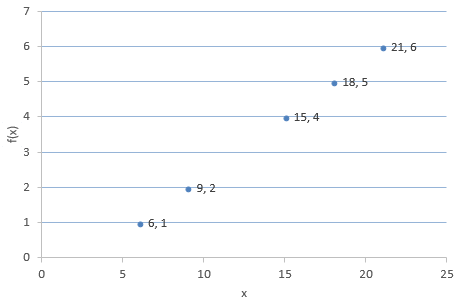Question 14.Let A = {4, 6, 8, 10 } and B = { 3, 4, 5, 6, 7 }. If f : A → B is defined by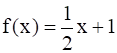then represent f by(i) an arrow diagram(ii) a set of ordered pairs(iii) a table.Answer:given A = {4, 6, 8, 10 }B = { 3, 4, 5, 6, 7 }.f : A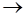B is defined by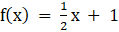so we can find the domain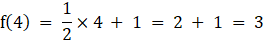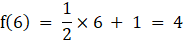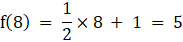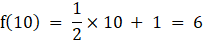(i) an arrow diagram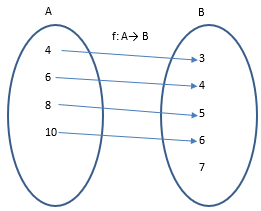(ii) a set of ordered pairsBasing on the available data we can write the function as asset of order pair as followsf(x) = {(4,3),(6,4),(8,5),(10,6)}(iii) a table.Using the data we have we can draw the tabel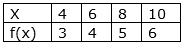Question 15.A function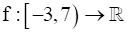is defined as follows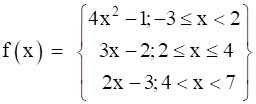Find (i) f(5) + f(6) (ii) f(1)- f(-3)(iii) f(-2h)- f(4) (iv)Answer:We are given,f: [-3,7) → ℝAnd the function given is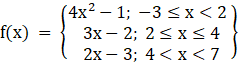(i) f(5) + f(6)Here it is clear that 5 and 6 lies between 4 and 7 so we have to use the function,f(x) = 2x - 3; 4 < x < 7So,f(5) = 2×5 - 3 = 10 - 3 = 7f(6) = 2×6 - 1 = 12 - 3 = 9∴ f(5) + f(6) = 7 + 9 = 16(ii) f(1) - f(-3)here its clear that 1 and -3 lies between the range of -3 ≤ x < 2 so we use the functionf(x) = 4x2 - 1⇒ f(1) = 4(1)2-1 = 4-1 = 3⇒ f(-3) = 4(-3)2-1 = 4×9 - 1 = 35So f(1) - f(-3)= 3 - 35= -32 (iii) f(-2) - f(4)As, x = -2f(x) = 4x2 -1; -3 ≤ x <2⇒ f(-2) = 4×(-2)2-1= 4×4 - 1= 16 - 1= 15For, x = 4f(x) = 3x-2; 2 ≤ x ≤ 4⇒ f(4) = 3 × 4 - 2= 12 - 2= 10⇒ f(-2)- f(4) = 15-10 = 5(iv)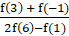Here the entire values ranges from -1 to 6 so the range will lie between -3≤x<2, 2≤ x<4 and 4<x<7Sof(3) = 3x - 2; 2≤ x<4= 3 × 3 – 2= 9 - 2 = 7f(-1) = 4x2 - 1: -3≤x<2= 4(-1)2-1= 4-1 = 3f(6) = 2x - 3; 4<x<7= 2 × 6-3= 12-3 = 9⇒ 2f(6) = 2 × 9 = 18f(1) = 4x2 - 1: -3≤x<2= 4(1)2 - 1= 4 - 1 = 3So the function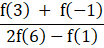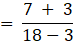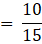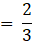Question 16.A function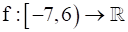is defined as follows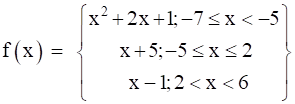(i) 2f(-4) + 3f(2)(ii) f (- 7) - f (- 3)(iii)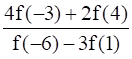Answer:We are given,f: [-7,6) → ℝ[-7,6) = {x ∈ A: -7≤x<6}= {-7, -6, -5, -4,-3, -2, -1, 0, 1, 2, 3, 4, 5}And the function given are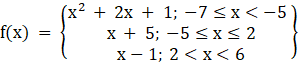(i) 2f(-4) + 3f(2)Here the function lies between -5<x<2Sof(x) = x + 5f(-4) = -4 + 5= 1f(2) = 2 + 5= 7∴ 2f(-4) = 2 × 1 = 2∴ 3f(2) = 3× 7 = 21So 2f(-4) + 3f(2)= 2 + 21 = 23(ii) f (- 7) - f (- 3)here the function lies between -7≤x<-5 and -5≤ x < 2so f(x) = x2 + 2x + 1⇒ f(-7) = (-7)2 + 2×(-7) + 1= 49-14 + 1= 36Similarly f(x) = x + 5⇒ f(-3) = -3 + 5= 2∴ f (- 7) - f (- 3)= 36 -2= 34(iii).For the above statement the function lies between-7≤ x <-5 for f(-6)-5≤ x<2 for for f(-3) and f(1)2<x<6 for f(4)at f(x) = x2 + 2x + 1 ; -7≤ x <-5⇒ f(-6) = (-6)2 + 2(-6) + 1= 36-12 + 1 = 25At f(x) = x + 5; -5≤ x<2 for⇒ f(-3) = -3 + 5 = 2⇒ 4f(-3) = 4 × 2 = 8Similarly,f(1) = 1 + 5 = 6⇒ 3f(1) = 3 × 6 = 18At f(x) = x-1; 2<x<6⇒ f(4) = 4-1 = 3⇒ 2f(4) = 2× 3 = 6So the function given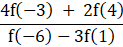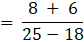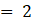PDF FILE TO YOUR EMAIL IMMEDIATELY PURCHASE NOTES & PAPER SOLUTION. @ Rs. 50/- each (GST extra)

HINDI ENTIRE PAPER SOLUTION

MARATHI PAPER SOLUTION

SSC MATHS I PAPER SOLUTION

SSC MATHS II PAPER SOLUTION

SSC SCIENCE I PAPER SOLUTION

SSC SCIENCE II PAPER SOLUTION

SSC ENGLISH PAPER SOLUTION

SSC & HSC ENGLISH WRITING SKILL

HSC ACCOUNTS NOTES

HSC OCM NOTES

HSC ECONOMICS NOTES

HSC SECRETARIAL PRACTICE NOTES

2019 Board Paper Solution

HSC ENGLISH SET A 2019 21st February, 2019

HSC ENGLISH SET B 2019 21st February, 2019

HSC ENGLISH SET C 2019 21st February, 2019

HSC ENGLISH SET D 2019 21st February, 2019

SECRETARIAL PRACTICE (S.P) 2019 25th February, 2019

HSC XII PHYSICS 2019 25th February, 2019

CHEMISTRY XII HSC SOLUTION 27th, February, 2019

OCM PAPER SOLUTION 2019 27th, February, 2019

HSC MATHS PAPER SOLUTION COMMERCE, 2nd March, 2019

HSC MATHS PAPER SOLUTION SCIENCE 2nd, March, 2019

SSC ENGLISH STD 10 5TH MARCH, 2019.

HSC XII ACCOUNTS 2019 6th March, 2019

HSC XII BIOLOGY 2019 6TH March, 2019

HSC XII ECONOMICS 9Th March 2019

SSC Maths I March 2019 Solution 10th Standard11th, March, 2019

SSC MATHS II MARCH 2019 SOLUTION 10TH STD.13th March, 2019

SSC SCIENCE I MARCH 2019 SOLUTION 10TH STD. 15th March, 2019.

SSC SCIENCE II MARCH 2019 SOLUTION 10TH STD. 18th March, 2019.

SSC SOCIAL SCIENCE I MARCH 2019 SOLUTION20th March, 2019

SSC SOCIAL SCIENCE II MARCH 2019 SOLUTION, 22nd March, 2019

XII CBSE - BOARD - MARCH - 2019 ENGLISH - QP + SOLUTIONS, 2nd March, 2019

HSC Maharashtra Board Papers 2020

(Std 12th English Medium)

HSC ECONOMICS MARCH 2020

HSC OCM MARCH 2020

HSC ACCOUNTS MARCH 2020

HSC S.P. MARCH 2020

HSC ENGLISH MARCH 2020

HSC HINDI MARCH 2020

HSC MARATHI MARCH 2020

HSC MATHS MARCH 2020

SSC Maharashtra Board Papers 2020

(Std 10th English Medium)

English MARCH 2020

HindI MARCH 2020

Hindi (Composite) MARCH 2020

Marathi MARCH 2020

Mathematics (Paper 1) MARCH 2020

Mathematics (Paper 2) MARCH 2020

Sanskrit MARCH 2020

Sanskrit (Composite) MARCH 2020

Science (Paper 1) MARCH 2020

Science (Paper 2)

MUST REMEMBER THINGS on the day of Exam

Are you prepared? for English Grammar in Board Exam.

Paper Presentation In Board Exam

How to Score Good Marks in SSC Board Exams

Tips To Score More Than 90% Marks In 12th Board Exam

How to write English exams?

How to prepare for board exam when less time is left

How to memorise what you learn for board exam

No. 1 Simple Hack, you can try out, in preparing for Board Exam

How to Study for CBSE Class 10 Board Exams Subject Wise Tips?

JEE Main 2020 Registration Process – Exam Pattern & Important Dates

NEET UG 2020 Registration Process Exam Pattern & Important Dates

How can One Prepare for two Competitive Exams at the same time?

8 Proven Tips to Handle Anxiety before Exams!